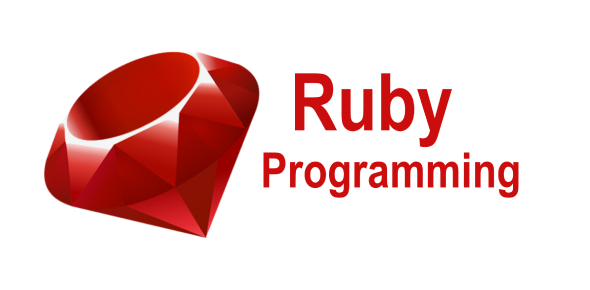12 Questions | Total Attempts: 1932SettingsRuby is an Object-Oriented and interpreted scripting language which executes instructions directly and freely, without compiling a program into machine-language instructions that have similar syntaxes like another programming language for eg. C and Java. This quiz has been made to test your skills and knowledge about the basic syntax of the Ruby programming language. So, let's try out the quiz. All the best!

• 1.
Ruby is what kind of programming language?
• A.

Strongly typed/Statically typed

• B.

Strongly typed/Dynamically typed

• C.

Weakly typed/Statically typed

• D.

Weakly typed/Dynamically typed

• E.

None of the above

• 2.
The following is a primitive type in Ruby
• A.

Integer

• B.

String

• C.

Float

• D.

Char

• E.

None of the Above

• 3.
Variables that start with two '@' symbols are what kind of variables in Ruby
• A.

Local variable

• B.

Global variable

• C.

Instance variable

• D.

Class variable

• E.

None of the above

• 4.
Variables that start with a capital letter are what kind of variables in Ruby
• A.

Local variable

• B.

Global variable

• C.

Instance variable

• D.

Class variable

• E.

None of the above

• 5.
Variables that start a dollar sign are what kind of variables in Ruby
• A.

Local variable

• B.

Global variable

• C.

Instance variable

• D.

Class variable

• E.

None of the above

• 6.
Ruby is an object-oriented general-purpose programming language.
• A.

True

• B.

False

• 7.
Which of the following is supported by Ruby?
• A.

• B.

Dynamic Type System

• C.

Automatic Memory Management

• D.

All of the Mentioned

• 8.
What is the output of the code? print "What's your first name?" first_name=gets.chomp a=first_name.capitalize first_name.capitalize! print "What's your last name?" last_name=gets.chomp b=last_name.capitalize last_name.capitalize puts "My name is #{first_name} #{last_name}"
• A.

Amil Jones

• B.

What’s your first name? amil What’s your last name? jones My name is Amil Jones

• C.

What’s your first name? amil What’s your last name? jones My name is Amil jones

• D.

None of the mentioned

• 9.
What is the output of the given code? x, y, z = 12, 36, 72 puts "The value of x is #{ x }." puts "The sum of x and y is #{ x + y }." puts "The average was #{ (x + y + z)/3 }."
• A.

12,48,40

• B.

Syntax error

• C.

The value of x is 12. The sum of x and y is 48. The average was 40.

• D.

None of the mentioned

• 10.
What does %{Learn ruby language} represents?
• A.

“Learn Ruby language”

• B.

“%{Learn Ruby language}”

• C.

” Learn Ruby language ”

• D.

None of the mentioned

• 11.
What does %x!ls! represents?
• A.

Same as back tick command output `ls`

• B.

Same as ‘x’

• C.

Same as “xls”

• D.

None of the mentioned

• 12.
Which of the following is the valid string method?
• A.

The .length method

• B.

The .upcase method

• C.

The .downcase method

• D.

The .irreverse method

Related TopicsBack to top